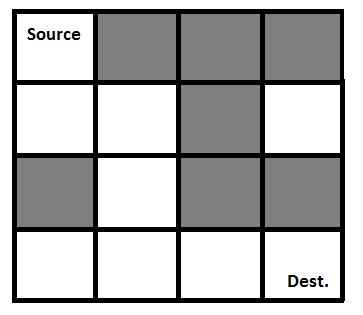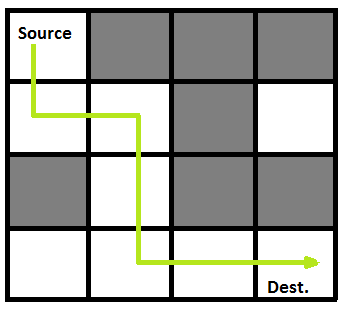Open in App
Not now

# Python Program for Rat in a Maze | Backtracking-2

• Difficulty Level : Expert
• Last Updated : 22 Apr, 2022

We have discussed Backtracking and Knight’s tour problem in Set 1. Let us discuss Rat in a Maze as another example problem that can be solved using Backtracking.

A Maze is given as N*N binary matrix of blocks where source block is the upper left most block i.e., maze and destination block is lower rightmost block i.e., maze[N-1][N-1]. A rat starts from source and has to reach the destination. The rat can move only in two directions: forward and down.
In the maze matrix, 0 means the block is a dead end and 1 means the block can be used in the path from source to destination. Note that this is a simple version of the typical Maze problem. For example, a more complex version can be that the rat can move in 4 directions and a more complex version can be with a limited number of moves.

Following is an example maze.

` Gray blocks are dead ends (value = 0). `Following is binary matrix representation of the above maze.

```                {1, 0, 0, 0}
{1, 1, 0, 1}
{0, 1, 0, 0}
{1, 1, 1, 1}
```

Following is a maze with highlighted solution path.Following is the solution matrix (output of program) for the above input matrix.

```                {1, 0, 0, 0}
{1, 1, 0, 0}
{0, 1, 0, 0}
{0, 1, 1, 1}
All entries in solution path are marked as 1.
```

## Python3

 `# Python3 program to solve Rat in a Maze ` `# problem using backtracking `   `# Maze size` `N ``=` `4`   `# A utility function to print solution matrix sol` `def` `printSolution( sol ):` `    `  `    ``for` `i ``in` `sol:` `        ``for` `j ``in` `i:` `            ``print``(``str``(j) ``+` `" "``, end ``=``"")` `        ``print``("")`   `# A utility function to check if x, y is valid` `# index for N * N Maze` `def` `isSafe( maze, x, y ):` `    `  `    ``if` `x >``=` `0` `and` `x < N ``and` `y >``=` `0` `and` `y < N ``and` `maze[x][y] ``=``=` `1``:` `        ``return` `True` `    `  `    ``return` `False`   `""" This function solves the Maze problem using Backtracking. ` `    ``It mainly uses solveMazeUtil() to solve the problem. It ` `    ``returns false if no path is possible, otherwise return ` `    ``true and prints the path in the form of 1s. Please note` `    ``that there may be more than one solutions, this function` `    ``prints one of the feasible solutions. """` `def` `solveMaze( maze ):` `    `  `    ``# Creating a 4 * 4 2-D list` `    ``sol ``=` `[ [ ``0` `for` `j ``in` `range``(``4``) ] ``for` `i ``in` `range``(``4``) ]` `    `  `    ``if` `solveMazeUtil(maze, ``0``, ``0``, sol) ``=``=` `False``:` `        ``print``(``"Solution doesn't exist"``);` `        ``return` `False` `    `  `    ``printSolution(sol)` `    ``return` `True` `    `  `# A recursive utility function to solve Maze problem` `def` `solveMazeUtil(maze, x, y, sol):` `    `  `    ``# if (x, y is goal) return True` `    ``if` `x ``=``=` `N ``-` `1` `and` `y ``=``=` `N ``-` `1``:` `        ``sol[x][y] ``=` `1` `        ``return` `True` `        `  `    ``# Check if maze[x][y] is valid` `    ``if` `isSafe(maze, x, y) ``=``=` `True``:` `        ``# mark x, y as part of solution path` `        ``sol[x][y] ``=` `1` `        `  `        ``# Move forward in x direction` `        ``if` `solveMazeUtil(maze, x ``+` `1``, y, sol) ``=``=` `True``:` `            ``return` `True` `            `  `        ``# If moving in x direction doesn't give solution ` `        ``# then Move down in y direction` `        ``if` `solveMazeUtil(maze, x, y ``+` `1``, sol) ``=``=` `True``:` `            ``return` `True` `        `  `        ``# If none of the above movements work then ` `        ``# BACKTRACK: unmark x, y as part of solution path` `        ``sol[x][y] ``=` `0` `        ``return` `False`   `# Driver program to test above function` `if` `__name__ ``=``=` `"__main__"``:` `    ``# Initialising the maze` `    ``maze ``=` `[ [``1``, ``0``, ``0``, ``0``],` `             ``[``1``, ``1``, ``0``, ``1``],` `             ``[``0``, ``1``, ``0``, ``0``],` `             ``[``1``, ``1``, ``1``, ``1``] ]` `             `  `    ``solveMaze(maze)`   `# This code is contributed by Shiv Shankar`

Output:

```1 0 0 0
1 1 0 0
0 1 0 0
0 1 1 1
```

Please refer complete article on Rat in a Maze | Backtracking-2 for more details!

My Personal Notes arrow_drop_up
Related Articles